# Symbolic Program Consistency Checking of Open MP Parallel

• Slides: 47
Download presentation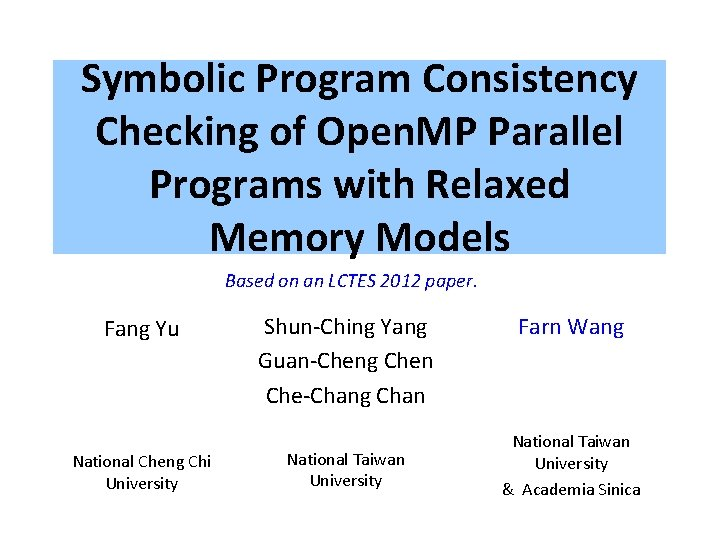Symbolic Program Consistency Checking of Open. MP Parallel Programs with Relaxed Memory Models Based on an LCTES 2012 paper. Fang Yu National Cheng Chi University Shun-Ching Yang Guan-Cheng Chen Che-Chang Chan National Taiwan University Farn Wang National Taiwan University & Academia Sinica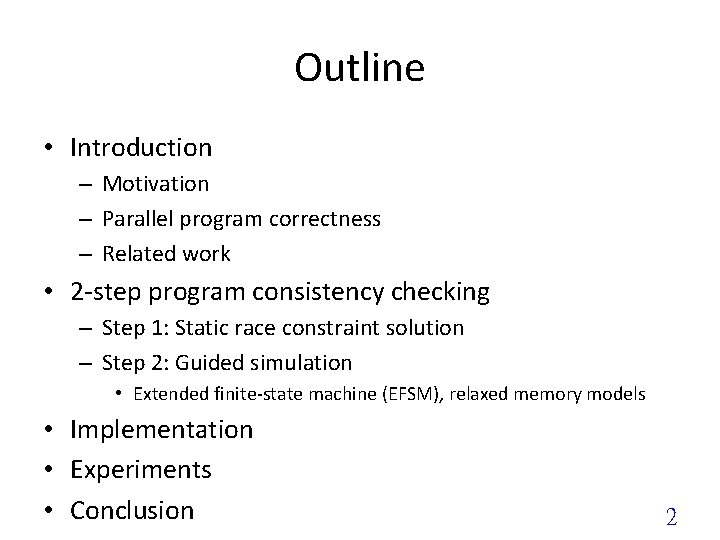Outline • Introduction – Motivation – Parallel program correctness – Related work • 2 -step program consistency checking – Step 1: Static race constraint solution – Step 2: Guided simulation • Extended finite-state machine (EFSM), relaxed memory models • Implementation • Experiments • Conclusion 2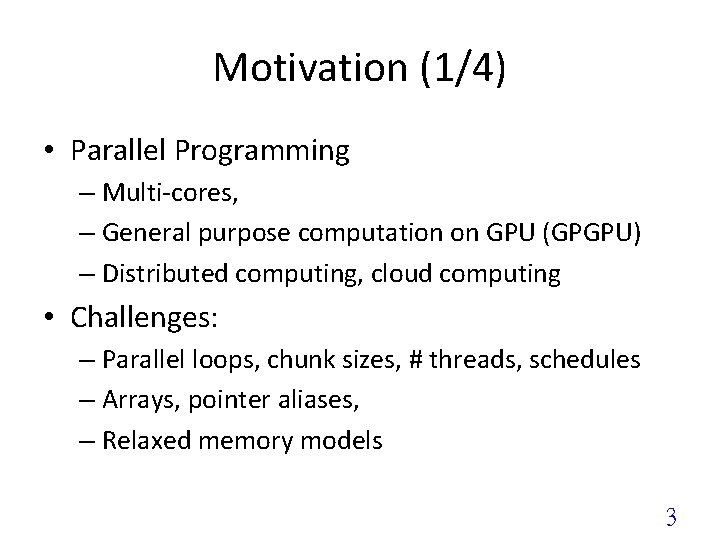Motivation (1/4) • Parallel Programming – Multi-cores, – General purpose computation on GPU (GPGPU) – Distributed computing, cloud computing • Challenges: – Parallel loops, chunk sizes, # threads, schedules – Arrays, pointer aliases, – Relaxed memory models 3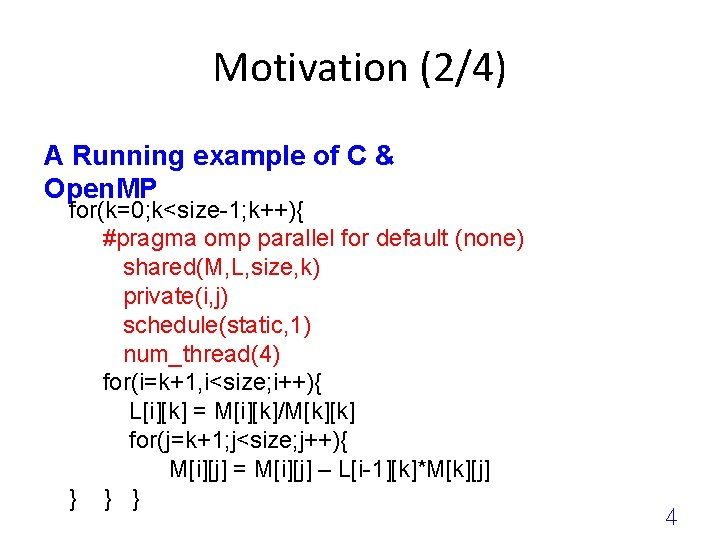Motivation (2/4) A Running example of C & Open. MP for(k=0; k<size-1; k++){ #pragma omp parallel for default (none) shared(M, L, size, k) private(i, j) schedule(static, 1) num_thread(4) for(i=k+1, i<size; i++){ L[i][k] = M[i][k]/M[k][k] for(j=k+1; j<size; j++){ M[i][j] = M[i][j] – L[i-1][k]*M[k][j] } } } 4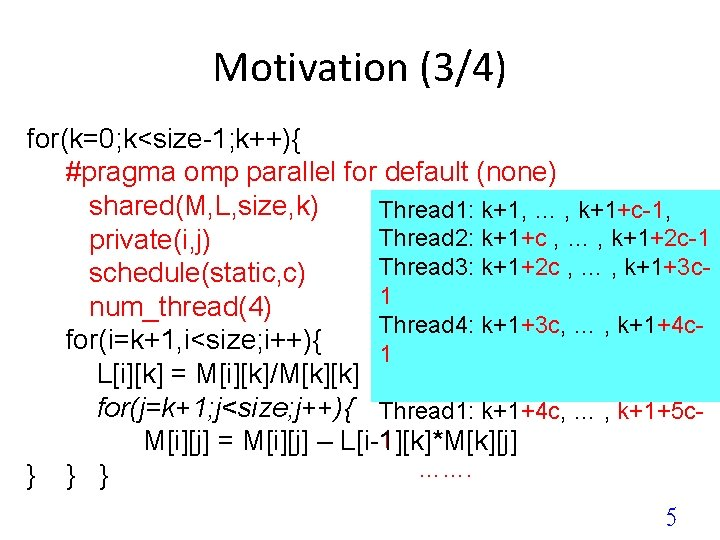Motivation (3/4) for(k=0; k<size-1; k++){ #pragma omp parallel for default (none) shared(M, L, size, k) Thread 1: k+1, … , k+1+c-1, Thread 2: k+1+c , … , k+1+2 c-1 private(i, j) Thread 3: k+1+2 c , … , k+1+3 cschedule(static, c) 1 num_thread(4) Thread 4: k+1+3 c, … , k+1+4 cfor(i=k+1, i<size; i++){ 1 L[i][k] = M[i][k]/M[k][k] for(j=k+1; j<size; j++){ Thread 1: k+1+4 c, … , k+1+5 c 1 M[i][j] = M[i][j] – L[i-1][k]*M[k][j] ……. } } } 5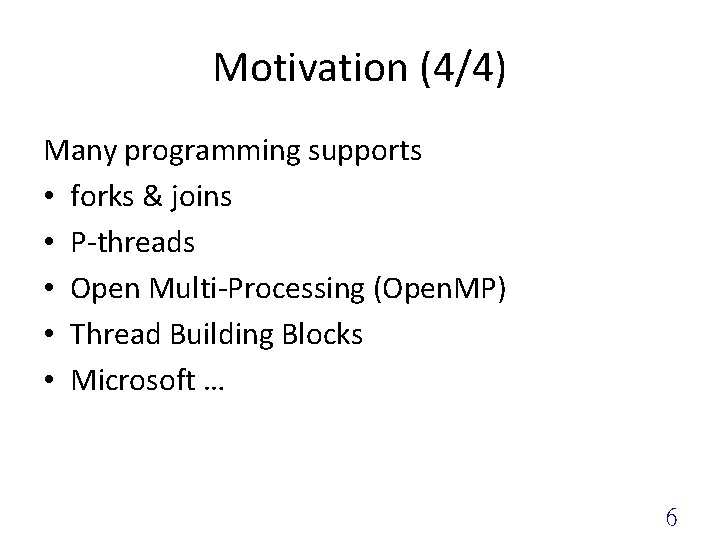Motivation (4/4) Many programming supports • forks & joins • P-threads • Open Multi-Processing (Open. MP) • Thread Building Blocks • Microsoft … 6Parallel Program Correctness (1/4) Program level, what users care about • Determinism: – For all input, all executions yield the same output. • Consistency: – All executions yield the same output as the sequential execution. • Race-freedom: – Parallel executions do not yield different results. All seemingly equivalent at program level. • unless sequential execution is not a parallel execution. 7Parallel Program Correctness (2/4) parallel for parallel while parallel for • Checking the correctness property of each parallel region (PR) • Correctness at PRs correctness of the program 8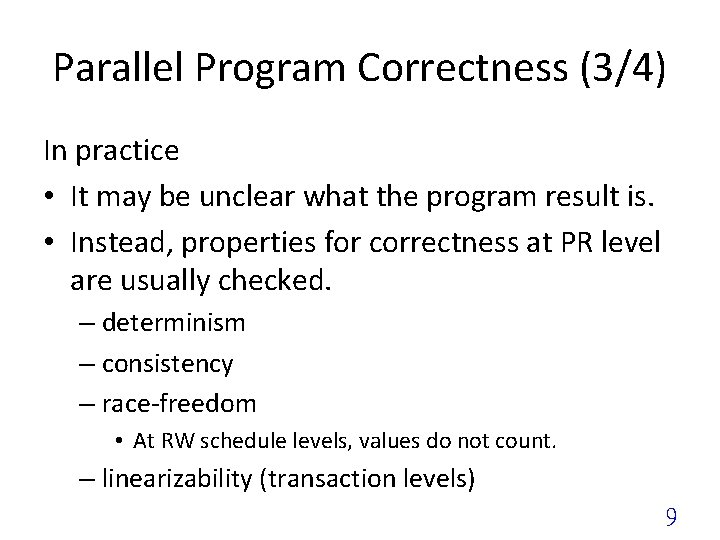Parallel Program Correctness (3/4) In practice • It may be unclear what the program result is. • Instead, properties for correctness at PR level are usually checked. – determinism – consistency – race-freedom • At RW schedule levels, values do not count. – linearizability (transaction levels) 9Parallel Program Correctness (4/4) Linearizability (Transaction level) race-freedom (PR RW level) determinism (PR level) = consistency (PR level) race-freedom (program level) = determinism (program level) = consistency (program level) program correctness 10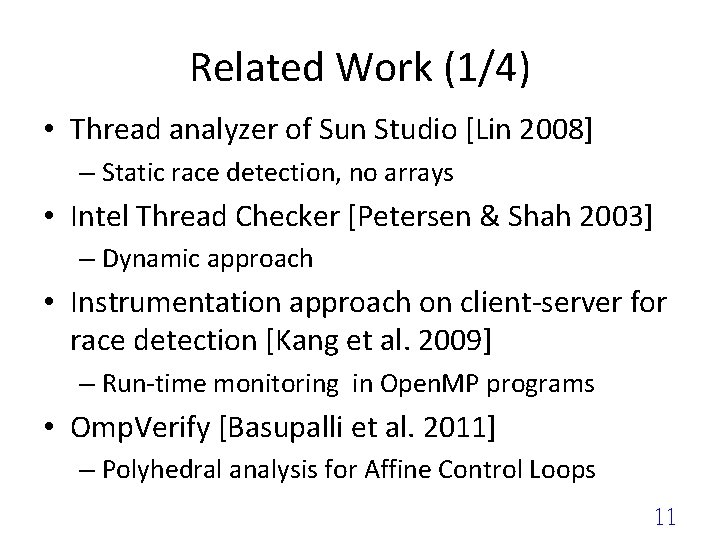Related Work (1/4) • Thread analyzer of Sun Studio [Lin 2008] – Static race detection, no arrays • Intel Thread Checker [Petersen & Shah 2003] – Dynamic approach • Instrumentation approach on client-server for race detection [Kang et al. 2009] – Run-time monitoring in Open. MP programs • Omp. Verify [Basupalli et al. 2011] – Polyhedral analysis for Affine Control Loops 11Related Work in PLDI 2012 (2/4) no simulation as the 2 nd step • Detect races via liquid effects [Kawaguchi, Rondon, Bakst, Jhala] – type inferencing for precise race detection. – no arrays. • Speculative Linearizability [Guerraoui, Kuncak, Losa] • Reasoning about Relaxed Programs [Carbin, Kim, Misailovic, Rinard] • Parallelizing Top-Down Interprocedural Analysis [Albarghouthi, Kumar, Nori, Rajamani] 12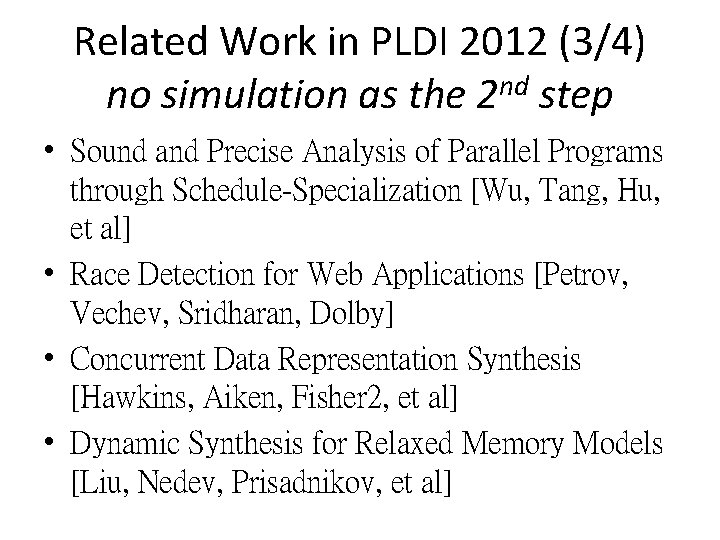Related Work in PLDI 2012 (3/4) no simulation as the 2 nd step • Sound and Precise Analysis of Parallel Programs through Schedule-Specialization [Wu, Tang, Hu, et al] • Race Detection for Web Applications [Petrov, Vechev, Sridharan, Dolby] • Concurrent Data Representation Synthesis [Hawkins, Aiken, Fisher 2, et al] • Dynamic Synthesis for Relaxed Memory Models [Liu, Nedev, Prisadnikov, et al]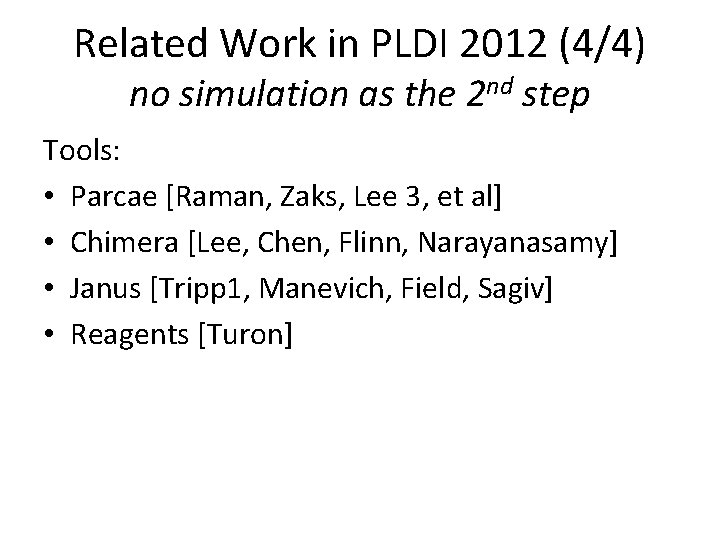Related Work in PLDI 2012 (4/4) no simulation as the 2 nd step Tools: • Parcae [Raman, Zaks, Lee 3, et al] • Chimera [Lee, Chen, Flinn, Narayanasamy] • Janus [Tripp 1, Manevich, Field, Sagiv] • Reagents [Turon]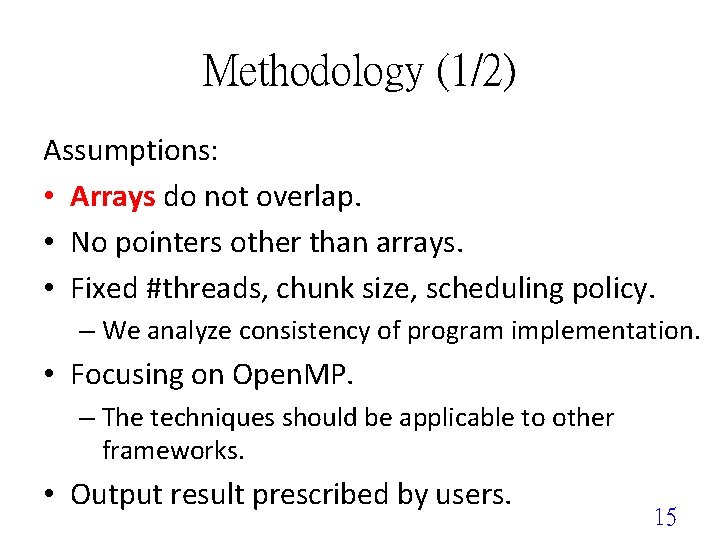Methodology (1/2) Assumptions: • Arrays do not overlap. • No pointers other than arrays. • Fixed #threads, chunk size, scheduling policy. – We analyze consistency of program implementation. • Focusing on Open. MP. – The techniques should be applicable to other frameworks. • Output result prescribed by users. 15Why Open. MP ? • Complicate enough • Practical enough – Parallelizes programs automatically; – Is an industry standard of application programming interface (API); – Is supported by Sun Studio, Intel Parallel Studio, Visual C++, GNU Compiler Collection (GCC). 16Methodology (2/2) 2 -step program consistency checking. Program Consistency checking Potential race analysis at PR level Potential race report Guided simulation for program consistency violations end 17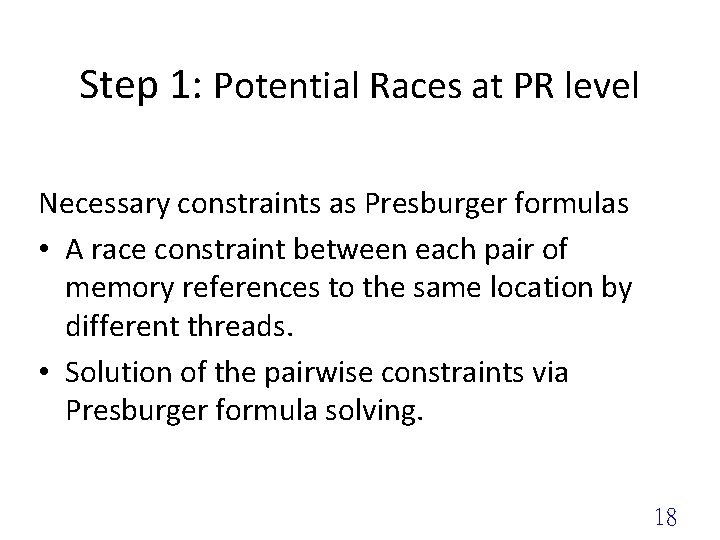Step 1: Potential Races at PR level Necessary constraints as Presburger formulas • A race constraint between each pair of memory references to the same location by different threads. • Solution of the pairwise constraints via Presburger formula solving. 18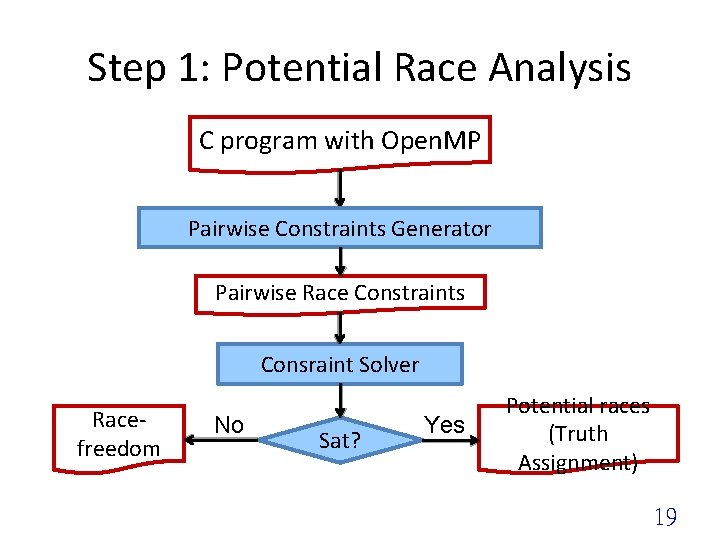Step 1: Potential Race Analysis C program with Open. MP Pairwise Constraints Generator Pairwise Race Constraints Consraint Solver Racefreedom No Sat? Yes Potential races (Truth Assignment) 19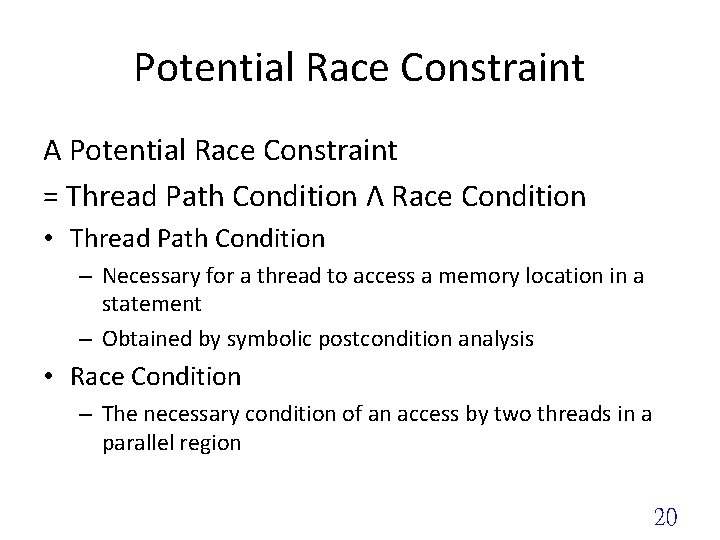Potential Race Constraint A Potential Race Constraint = Thread Path Condition Λ Race Condition • Thread Path Condition – Necessary for a thread to access a memory location in a statement – Obtained by symbolic postcondition analysis • Race Condition – The necessary condition of an access by two threads in a parallel region 20Running example for(k=0; k<size-1; k++){ #pragma omp parallel for default (none) shared(M, L, size, k) Thread 1: k+1, … , k+1+c-1, Thread 2: k+1+c , … , k+1+2 c-1 private(i, j) Thread 3: k+1+2 c , … , k+1+3 cschedule(static, c) 1 num_thread(4) Thread 4: k+1+3 c, … , k+1+4 cfor(i=k+1, i<size; i++){ 1 L[i][k] = M[i][k]/M[k][k] for(j=k+1; j<size; j++){ Thread 1: k+1+4 c, … , k+1+5 c 1 M[i][j] = M[i][j] – L[i-1][k]*M[k][j] ……. } } } 21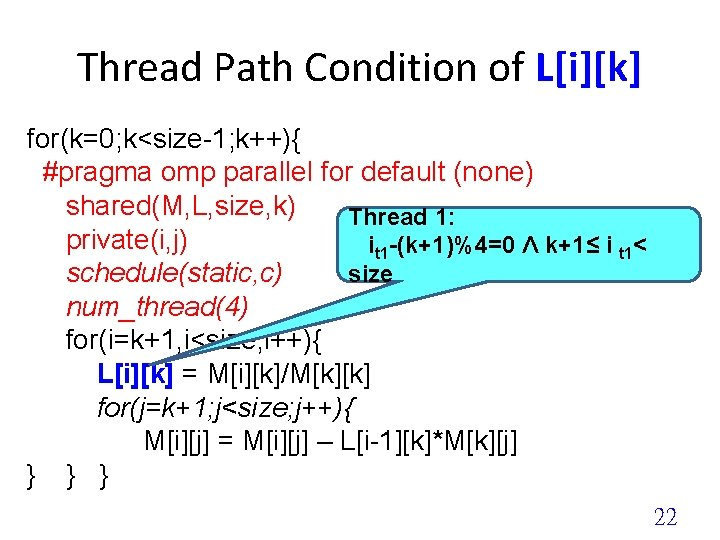Thread Path Condition of L[i][k] for(k=0; k<size-1; k++){ #pragma omp parallel for default (none) shared(M, L, size, k) Thread 1: private(i, j) it 1 -(k+1)%4=0 Λ k+1≤ i t 1< schedule(static, c) size num_thread(4) for(i=k+1, i<size; i++){ L[i][k] = M[i][k]/M[k][k] for(j=k+1; j<size; j++){ M[i][j] = M[i][j] – L[i-1][k]*M[k][j] } } } 22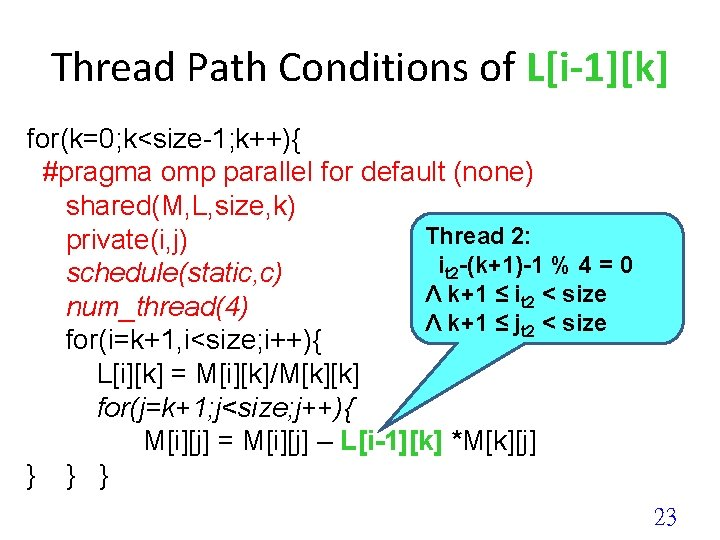Thread Path Conditions of L[i-1][k] for(k=0; k<size-1; k++){ #pragma omp parallel for default (none) shared(M, L, size, k) Thread 2: private(i, j) it 2 -(k+1)-1 % 4 = 0 schedule(static, c) Λ k+1 ≤ it 2 < size num_thread(4) Λ k+1 ≤ jt 2 < size for(i=k+1, i<size; i++){ L[i][k] = M[i][k]/M[k][k] for(j=k+1; j<size; j++){ M[i][j] = M[i][j] – L[i-1][k] *M[k][j] } } } 23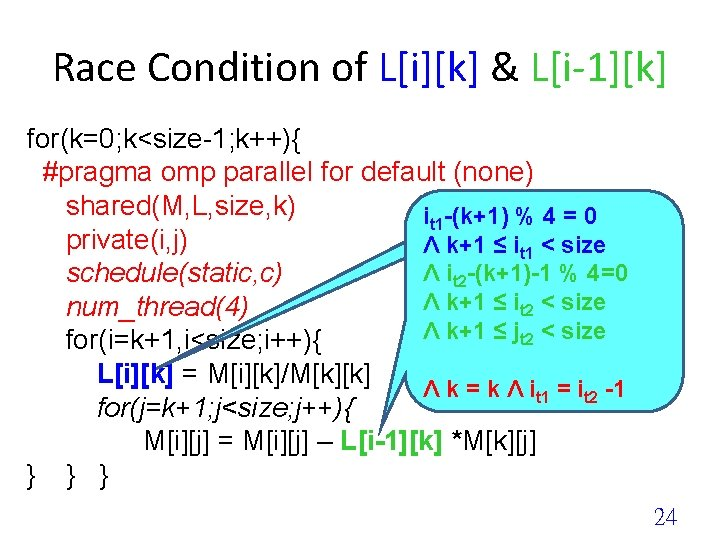Race Condition of L[i][k] & L[i-1][k] for(k=0; k<size-1; k++){ #pragma omp parallel for default (none) shared(M, L, size, k) it 1 -(k+1) % 4 = 0 private(i, j) Λ k+1 ≤ it 1 < size Λ it 2 -(k+1)-1 % 4=0 schedule(static, c) Λ k+1 ≤ it 2 < size num_thread(4) Λ k+1 ≤ jt 2 < size for(i=k+1, i<size; i++){ L[i][k] = M[i][k]/M[k][k] Λ k = k Λ it 1 = it 2 -1 for(j=k+1; j<size; j++){ M[i][j] = M[i][j] – L[i-1][k] *M[k][j] } } } 24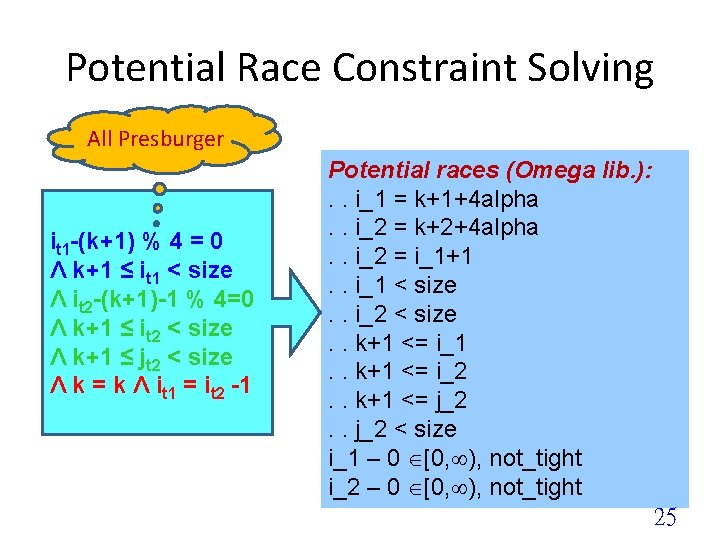Potential Race Constraint Solving All Presburger it 1 -(k+1) % 4 = 0 Λ k+1 ≤ it 1 < size Λ it 2 -(k+1)-1 % 4=0 Λ k+1 ≤ it 2 < size Λ k+1 ≤ jt 2 < size Λ k = k Λ it 1 = it 2 -1 Potential races (Omega lib. ): . . i_1 = k+1+4 alpha. . i_2 = k+2+4 alpha. . i_2 = i_1+1. . i_1 < size. . i_2 < size. . k+1 <= i_1. . k+1 <= i_2. . k+1 <= j_2. . j_2 < size i_1 – 0 [0, ), not_tight i_2 – 0 [0, ), not_tight 25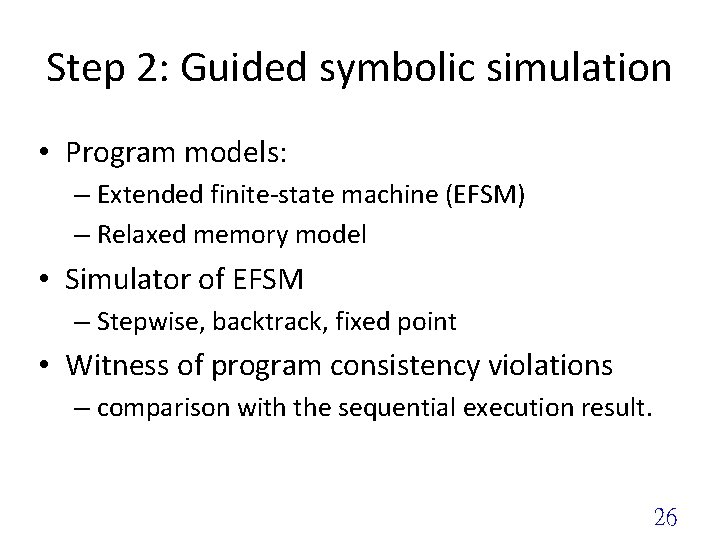Step 2: Guided symbolic simulation • Program models: – Extended finite-state machine (EFSM) – Relaxed memory model • Simulator of EFSM – Stepwise, backtrack, fixed point • Witness of program consistency violations – comparison with the sequential execution result. 26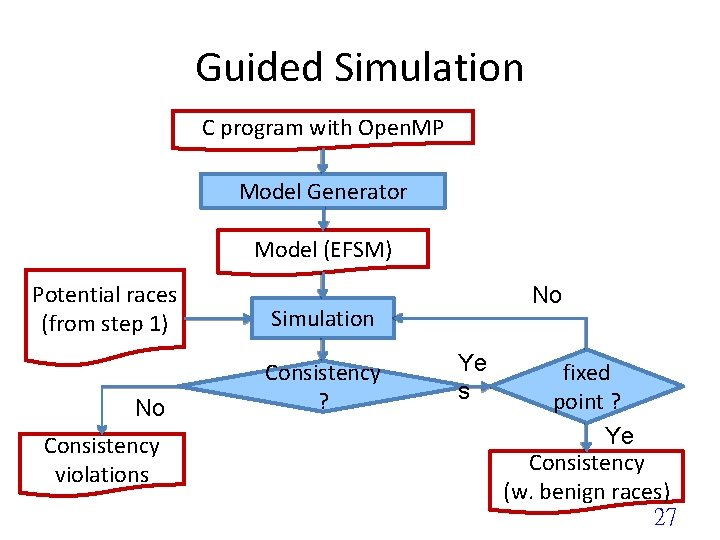Guided Simulation C program with Open. MP Model Generator Model (EFSM) Potential races (from step 1) No Consistency violations No Simulation Consistency ? Ye s fixed point ? Ye Consistency s (w. benign races) 27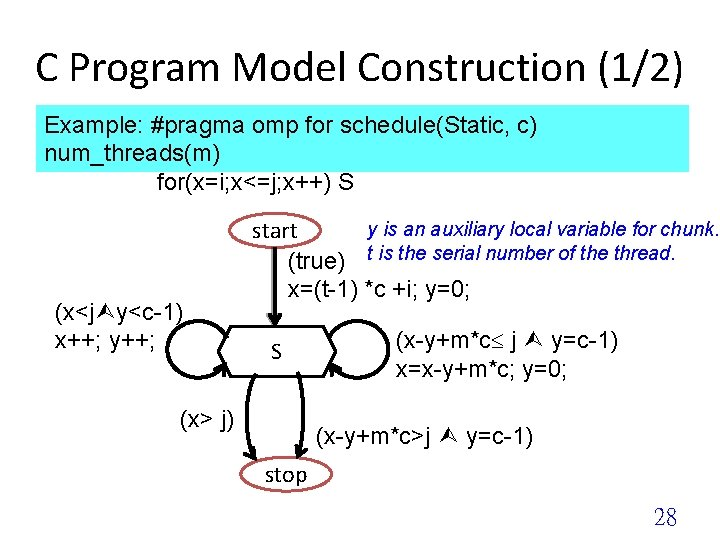C Program Model Construction (1/2) Example: #pragma omp for schedule(Static, c) num_threads(m) for(x=i; x<=j; x++) S (x<j y<c-1) x++; y++; y is an auxiliary local variable for chunk. start (true) t is the serial number of the thread. x=(t-1) *c +i; y=0; S (x> j) (x-y+m*c j y=c-1) x=x-y+m*c; y=0; (x-y+m*c>j y=c-1) stop 28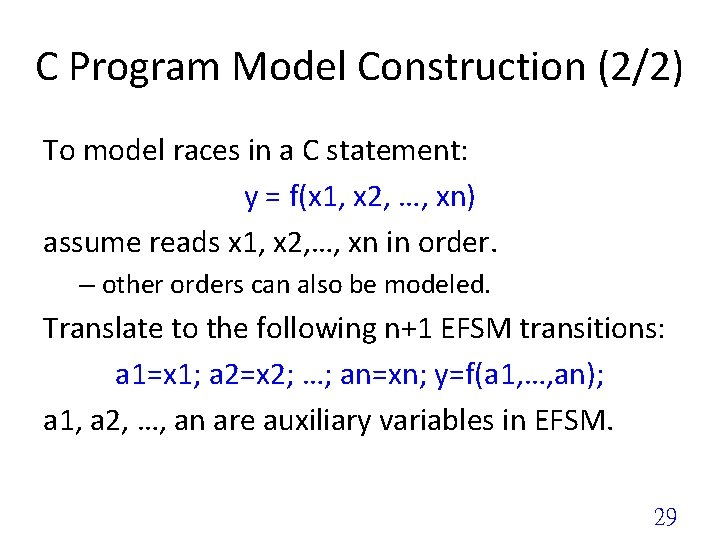C Program Model Construction (2/2) To model races in a C statement: y = f(x 1, x 2, …, xn) assume reads x 1, x 2, …, xn in order. – other orders can also be modeled. Translate to the following n+1 EFSM transitions: a 1=x 1; a 2=x 2; …; an=xn; y=f(a 1, …, an); a 1, a 2, …, an are auxiliary variables in EFSM. 29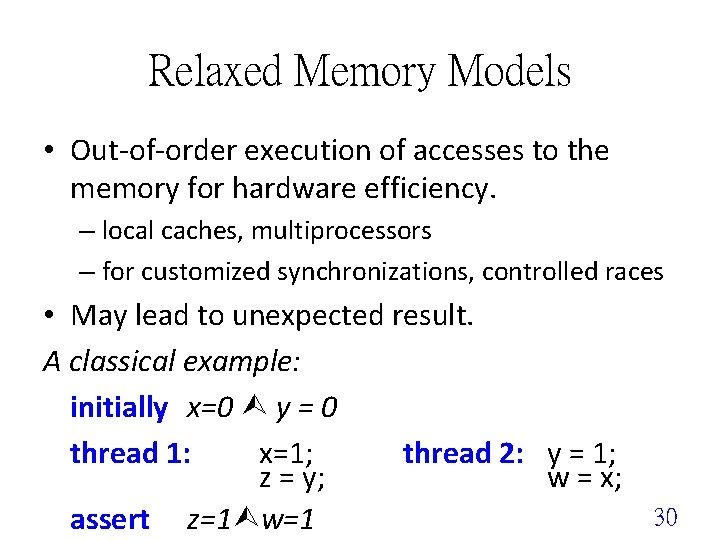Relaxed Memory Models • Out-of-order execution of accesses to the memory for hardware efficiency. – local caches, multiprocessors – for customized synchronizations, controlled races • May lead to unexpected result. A classical example: initially x=0 y = 0 thread 1: x=1; thread 2: y = 1; z = y; w = x; assert z=1 w=1 30Relaxed Memory Models A classical example: initially x=0 y = 0 thread 1: x=1; z=y; cache 1 thread 2: y=1; w=x; assert cache 2 z=1 w=1 store load memory x. c 1=1 y. c 1=1 load(w. c 2, x) load(z. c 1, y) store(x. c 1) x=x. c 1 store(y. c 2) y=y. c 2 31Relaxed Memory Models Total store order (TSO) • From SPARC • Adapted to Intel 80 x 86 series • Description: – Local reads can use pending writes in the local store. • Problem: Peer reads are not aware of the local pending writes. – Local stores must be FIFO. 32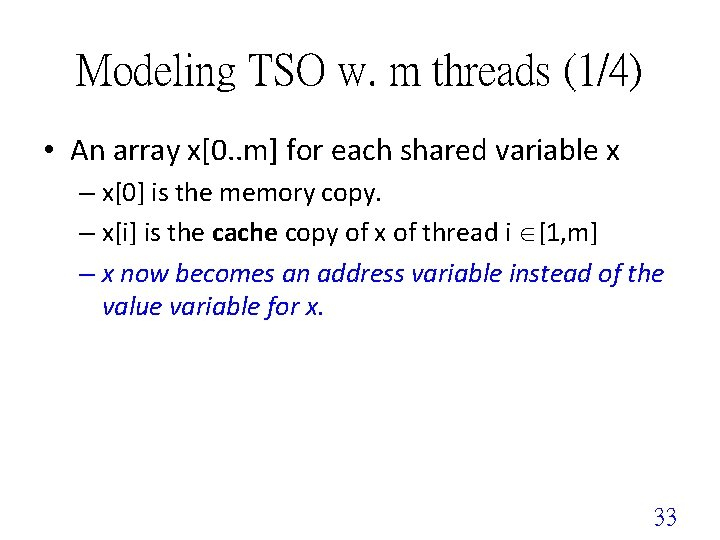Modeling TSO w. m threads (1/4) • An array x[0. . m] for each shared variable x – x is the memory copy. – x[i] is the cache copy of x of thread i [1, m] – x now becomes an address variable instead of the value variable for x. 33Modeling TSO w. m threads (2/4) • An arrays ls[0. . n] of objects for load-store (LS) buffer of size n+1. – ls_st[k]: status of load-store buffer cell k • 0: not used, 1: load, 2: store – ls_th[k]: thread that use load-store buffer cell k. – ls_dst[k], ls_src[k]: destination and source addresses – ls_value: value to store Purely for convenience. Can be changed to m load-store buffers for each thread. Need know mappings from threads to cores 34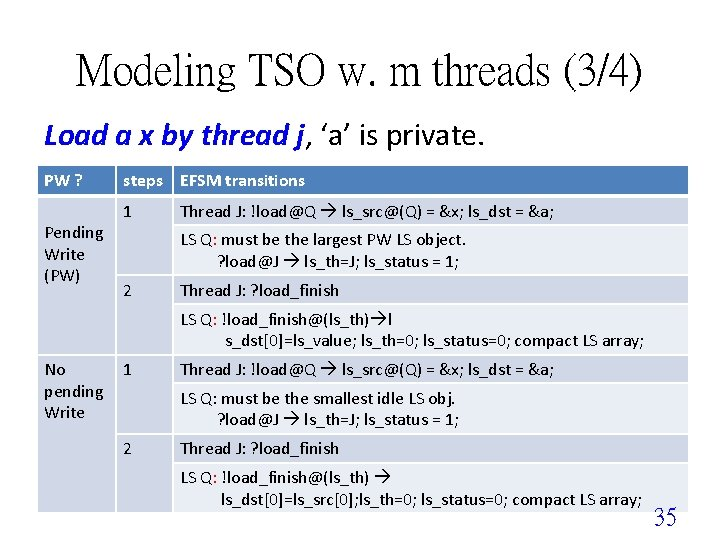Modeling TSO w. m threads (3/4) Load a x by thread j, ‘a’ is private. PW ? Pending Write (PW) steps EFSM transitions 1 Thread J: [email protected] [email protected](Q) = &x; ls_dst = &a; LS Q: must be the largest PW LS object. ? [email protected] ls_th=J; ls_status = 1; 2 Thread J: ? load_finish LS Q: [email protected](ls_th) l s_dst=ls_value; ls_th=0; ls_status=0; compact LS array; No 1 pending Write 2 Thread J: [email protected] [email protected](Q) = &x; ls_dst = &a; LS Q: must be the smallest idle LS obj. ? [email protected] ls_th=J; ls_status = 1; Thread J: ? load_finish LS Q: [email protected](ls_th) ls_dst=ls_src; ls_th=0; ls_status=0; compact LS array; 35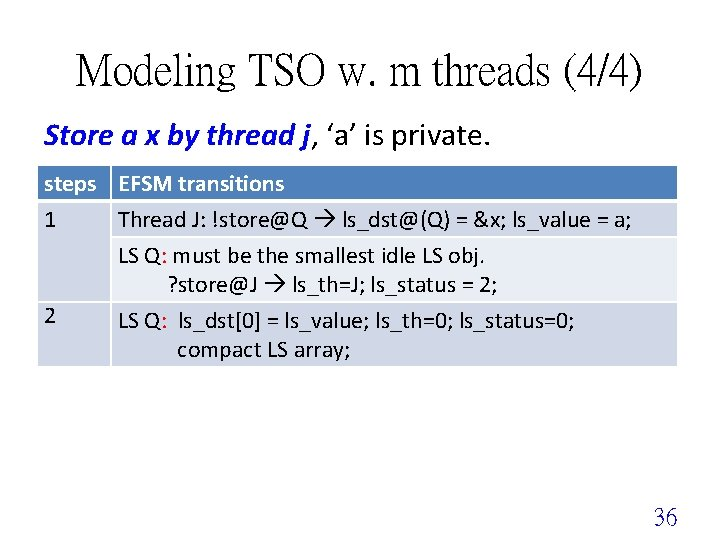Modeling TSO w. m threads (4/4) Store a x by thread j, ‘a’ is private. steps EFSM transitions 1 Thread J: [email protected] [email protected](Q) = &x; ls_value = a; LS Q: must be the smallest idle LS obj. ? [email protected] ls_th=J; ls_status = 2; 2 LS Q: ls_dst = ls_value; ls_th=0; ls_status=0; compact LS array; 36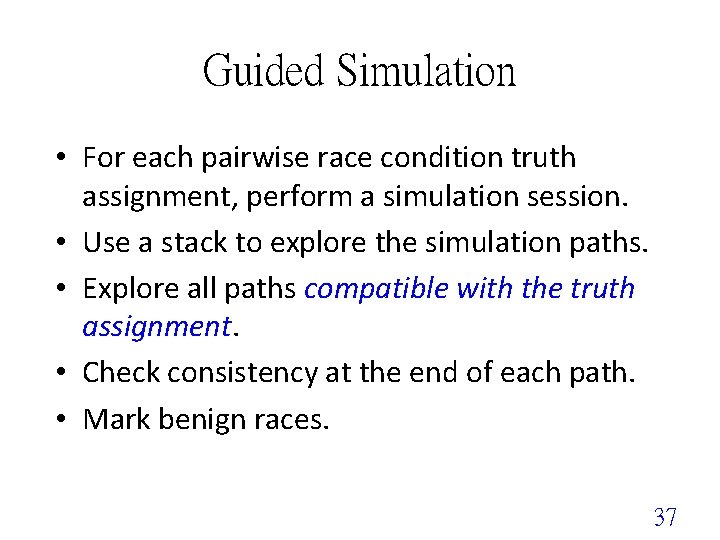Guided Simulation • For each pairwise race condition truth assignment, perform a simulation session. • Use a stack to explore the simulation paths. • Explore all paths compatible with the truth assignment. • Check consistency at the end of each path. • Mark benign races. 37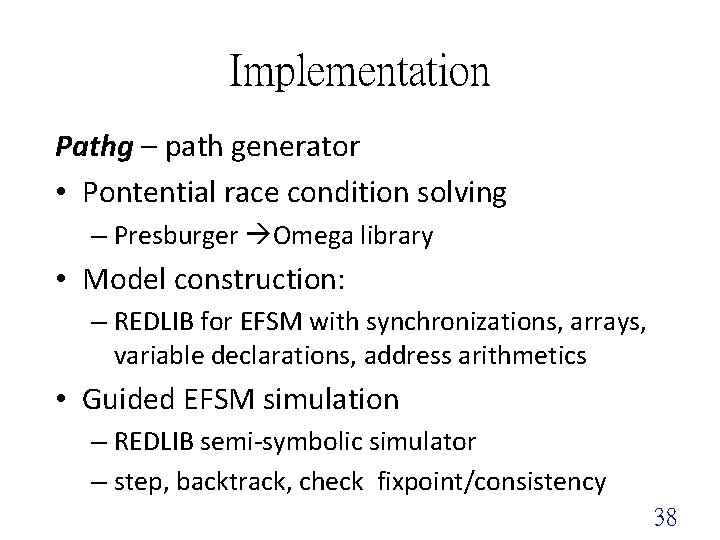Implementation Pathg – path generator • Pontential race condition solving – Presburger Omega library • Model construction: – REDLIB for EFSM with synchronizations, arrays, variable declarations, address arithmetics • Guided EFSM simulation – REDLIB semi-symbolic simulator – step, backtrack, check fixpoint/consistency 38Implementation Guided Symbolic Simulation Sequential execution(Golden model) Master Thread Parallel Task 1 Parallel Task 2 Parallel Task 3 Master Thread Memory Accessing Sequence Read: L Write: L. . output Guided Multi-Threaded Simulation Memory Accessing Sequence Master Thread Read: L Write: L. . output Parallel Task 1 Parallel Task 2 Parallel Task 3 Master Thread 39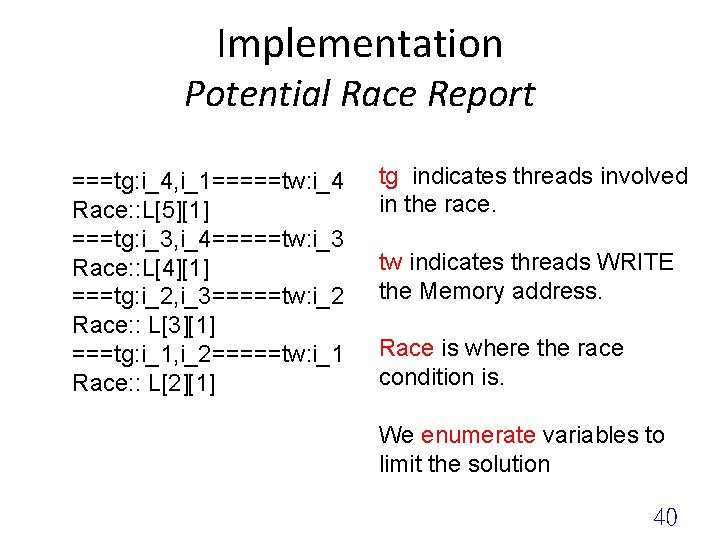Implementation Potential Race Report ===tg: i_4, i_1=====tw: i_4 Race: : L ===tg: i_3, i_4=====tw: i_3 Race: : L ===tg: i_2, i_3=====tw: i_2 Race: : L ===tg: i_1, i_2=====tw: i_1 Race: : L tg indicates threads involved in the race. tw indicates threads WRITE the Memory address. Race is where the race condition is. We enumerate variables to limit the solution 40Experiments • Environment – Ubuntu 9. 10 64 bit – i 5 -760 2. 8 GHz and 2 GB RAM • Benchmarks – Open. MP Source Code Repository (Omp. SCR) – NAS Parallel Benchmarks (NPB) 41Constraint Solving of Omp. SCR ß Bug v 1: Races manually introduced (between any two threads dealing with the consecutive iterations) ß Bug v 2: Rare races introduced (only between two specific threads on a particular share memory) ß Fixed: A barrier statement manually inserted (remove the race in Bug v 2) Benchmark Original Bug v 1 Bug v 2 Fixed #Const. #Sat Time c_lu. c 71 0 0. 18 s 629 29 1. 810 s 935 30 4. 110 s 935 0 5. 15 s c_ja 01. c 95 0 0. 39 s 95 8 0. 42 s 155 1 0. 75 s 95 0 0. 77 s c_ja 02. c 95 0 0. 03 s 95 8 0. 35 s 155 1 0. 67 s 95 0 1. 03 s c_loop. A. c 17 0 0. 04 s 47 4 0. 07 s 95 1 0. 32 s 17 0 0. 84 s c_loop. B. c 17 0 0. 03 s 29 4 0. 08 s 95 1 0. 15 s 17 0 1. 13 s c_md. c 65 0 0. 25 s 77 4 0. 30 s 131 1 0. 53 s 65 0 1. 25 s 42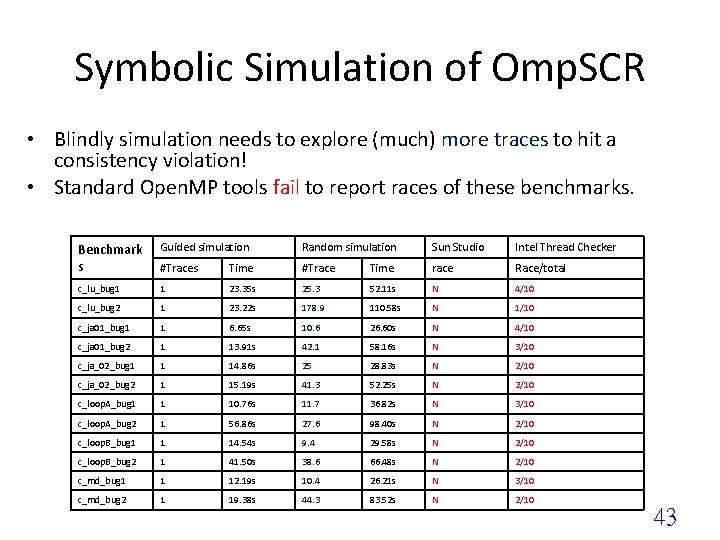Symbolic Simulation of Omp. SCR • Blindly simulation needs to explore (much) more traces to hit a consistency violation! • Standard Open. MP tools fail to report races of these benchmarks. Benchmark s Guided simulation Random simulation Sun Studio Intel Thread Checker #Traces Time #Trace Time race Race/total c_lu_bug 1 1 23. 35 s 25. 3 52. 11 s N 4/10 c_lu_bug 2 1 23. 22 s 178. 9 110. 58 s N 1/10 c_ja 01_bug 1 1 6. 65 s 10. 6 26. 60 s N 4/10 c_ja 01_bug 2 1 13. 91 s 42. 1 58. 16 s N 3/10 c_ja_02_bug 1 1 14. 86 s 25 28. 83 s N 2/10 c_ja_02_bug 2 1 15. 19 s 41. 3 52. 25 s N 2/10 c_loop. A_bug 1 1 10. 76 s 11. 7 36. 82 s N 3/10 c_loop. A_bug 2 1 56. 86 s 27. 6 98. 40 s N 2/10 c_loop. B_bug 1 1 14. 54 s 9. 4 29. 58 s N 2/10 c_loop. B_bug 2 1 41. 50 s 38. 6 66. 48 s N 2/10 c_md_bug 1 1 12. 19 s 10. 4 26. 21 s N 3/10 c_md_bug 2 1 19. 38 s 44. 3 83. 52 s N 2/10 43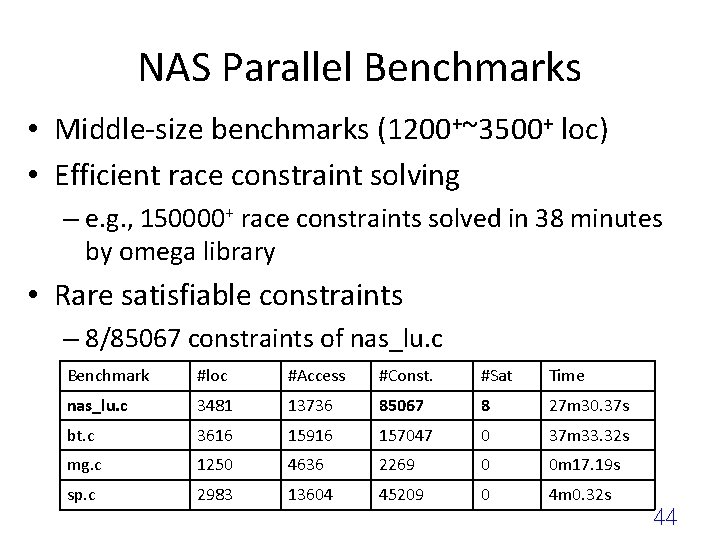NAS Parallel Benchmarks • Middle-size benchmarks (1200+~3500+ loc) • Efficient race constraint solving – e. g. , 150000+ race constraints solved in 38 minutes by omega library • Rare satisfiable constraints – 8/85067 constraints of nas_lu. c Benchmark #loc #Access #Const. #Sat Time nas_lu. c 3481 13736 85067 8 27 m 30. 37 s bt. c 3616 15916 157047 0 37 m 33. 32 s mg. c 1250 4636 2269 0 0 m 17. 19 s sp. c 2983 13604 45209 0 4 m 0. 32 s 44nas_lu. c • Slice the program to the segment of the paralleled region with satisfiable race conditions • Construct the symbolic model of the sliced segment: – 35 Modes (EFSM) – Reaching the fixed point without consistency violation after 205 steps and 16. 93 secs • Benign races – All of them are used as mutual exclusion semaphores – nas_lu. c is consistent 45Conclusion • Static analysis of program consistency – for real C/C++ program with Open. MP directives • Highly automated solution – Constraint solving – Symbolic simulation • • High precision: relaxed memory models High efficiency Extension to TBB, other memory models ? Partial order reduction ? 46Conclusion Symbolic approach for static consistency checking – Detect and identify races by solving race constraints (Presburger formulas) – Construct symbolic models and perform guided simulation with races – Support relaxed memory models – Find consistency violations effectively (when existing) 47